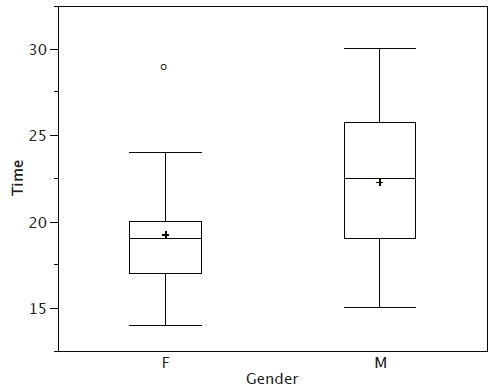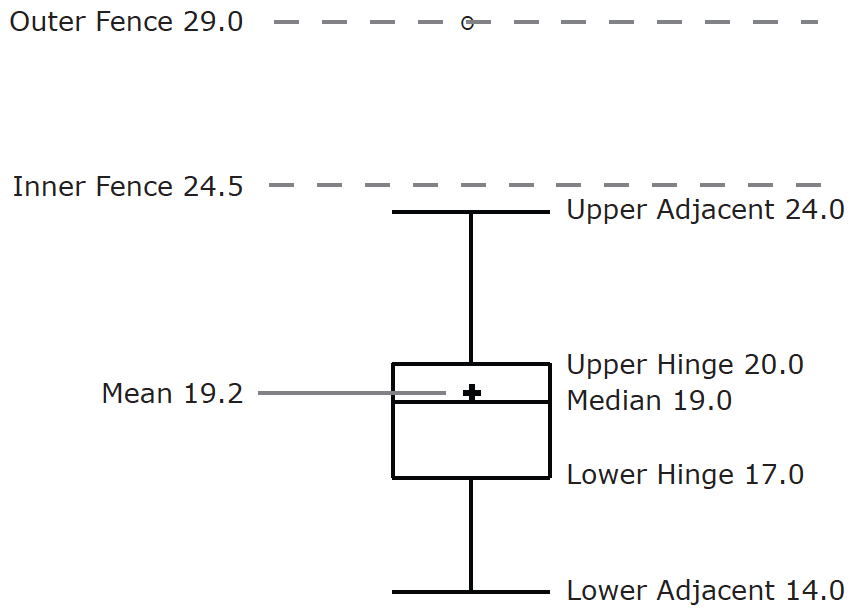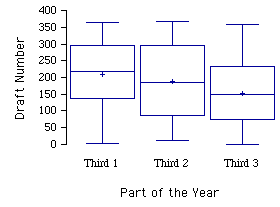# 2.6: Box Plots

•• Contributed by David Lane
• Associate Professor (Psychology, Statistics, and Management) at Rice University

Learning Objectives

• Define basic terms including hinges, H-spread, step, adjacent value, outside value, and far out value
• Create a box plot
• Create parallel box plots
• Determine whether a box plot is appropriate for a given data set

We have already discussed techniques for visually representing data (see histograms and frequency polygons). In this section, we present another important graph called a box plot. Box plots are useful for identifying outliers and for comparing distributions. We will explain box plots with the help of data from an in-class experiment. As part of the "Stroop Interference Case Study," students in introductory statistics were presented with a page containing $$30$$ colored rectangles. Their task was to name the colors as quickly as possible. Their times (in seconds) were recorded. We'll compare the scores for the $$16$$ men and $$31$$ women who participated in the experiment by making separate box plots for each gender. Such a display is said to involve parallel box plots.

There are several steps in constructing a box plot. The first relies on the $$25^{th},\; 50^{th},\; and\; 75^{th}$$ percentiles in the distribution of scores. Figure $$\PageIndex{1}$$shows how these three statistics are used. For each gender, we draw a box extending from the $$25^{th}$$ percentile to the $$75^{th}$$ percentile. The $$50^{th}$$ percentile is drawn inside the box. Therefore,

• the bottom of each box is the $$25^{th}$$ percentile,
• the top is the $$75^{th}$$ percentile,
• and the line in the middle is the $$50^{th}$$ percentile.

The data for the women in our sample are shown in Table $$\PageIndex{1}$$.

 14 17 18 19 20 21 29 15 17 18 19 20 22 16 17 18 19 20 23 16 17 18 20 20 24 17 18 18 20 21 24

For these data, the $$25^{th}$$ percentile is $$17$$, the $$50^{th}$$ percentile is $$19$$, and the $$75^{th}$$ percentile is $$20$$. For the men (whose data are not shown), the $$25^{th}$$ percentile is $$19$$, the $$50^{th}$$ percentile is $$22.5$$, and the $$75^{th}$$ percentile is $$25.5$$.Figure $$\PageIndex{1}$$: The first step in creating box plots

Before proceeding, the terminology in Table $$\PageIndex{2}$$ is helpful.

Table $$\PageIndex{2}$$: Box plot terms and values for women's times
Name Formula Value
Upper Hinge 75th Percentile 20
Lower Hinge 25th Percentile 17
H-Spread Upper Hinge - Lower Hinge 3
Upper Inner Fence Upper Hinge + 1 Step 24.5
Lower Inner Fence Lower Hinge - 1 Step 12.5
Upper Outer Fence Upper Hinge + 2 Steps 29
Lower Outer Fence Lower Hinge - 2 Steps 8
Upper Adjacent Largest value below Upper Inner Fence 24

Smallest value above Lower Inner Fence 14
Outside Value A value beyond an Inner Fence but not beyond an Outer Fence 29
Far Out Value A value beyond an Outer Fence None

Continuing with the box plots, we put "whiskers" above and below each box to give additional information about the spread of the data. Whiskers are vertical lines that end in a horizontal stroke. Whiskers are drawn from the upper and lower hinges to the upper and lower adjacent values ($$24$$ and $$14$$ for the women's data).Figure $$\PageIndex{2}$$: The box plots with the whiskers drawn

Although we don't draw whiskers all the way to outside or far out values, we still wish to represent them in our box plots. This is achieved by adding additional marks beyond the whiskers. Specifically, outside values are indicated by small "$$o's$$" and far out values are indicated by asterisks ($$\ast$$). In our data, there are no far out values and just one outside value. This outside value of $$29$$ is for the women and is shown in Figure $$\PageIndex{3}$$.Figure $$\PageIndex{3}$$: The box plots with the outside value shown

There is one more mark to include in box plots (although sometimes it is omitted). We indicate the mean score for a group by inserting a plus sign. Figure $$\PageIndex{4}$$ shows the result of adding means to our box plots.Figure $$\PageIndex{4}$$: The completed box plots

Figure $$\PageIndex{4}$$ provides a revealing summary of the data. Since half the scores in a distribution are between the hinges (recall that the hinges are the $$25^{th}$$ and $$75^{th}$$ percentiles), we see that half the women's times are between $$17$$ and $$20$$ seconds, whereas half the men's times are between $$19$$ and $$25.5$$. We also see that women generally named the colors faster than the men did, although one woman was slower than almost all of the men. Figure $$\PageIndex{5}$$ shows the box plot for the women's data with detailed labels.Figure $$\PageIndex{5}$$: The box plot for the women's data with detailed labels

Box plots provide basic information about a distribution. For example, a distribution with a positive skew would have a longer whisker in the positive direction than in the negative direction. A larger mean than median would also indicate a positive skew. Box plots are good at portraying extreme values and are especially good at showing differences between distributions. However, many of the details of a distribution are not revealed in a box plot, and to examine these details one should create a histogram and/or a stem and leaf display.

Here are some other examples of box plots:

Example $$\PageIndex{1}$$: Time to move the mouse over a target

The data come from a task in which the goal is to move a computer mouse to a target on the screen as fast as possible. On $$20$$ of the trials, the target was a small rectangle; on the other $$20$$, the target was a large rectangle. Time to reach the target was recorded on each trial. The box plots of the two distributions are shown below. You can see that although there is some overlap in times, it generally took longer to move the mouse to the small target than to the large one.Figure $$\PageIndex{6}$$: Target Box Plots

Example $$\PageIndex{2}$$: Draft lottery

In $$1969$$ the war in Vietnam was at its height. An agency called the Selective Service was charged with finding a fair procedure to determine which young men would be conscripted ("drafted") into the U.S. military. The procedure was supposed to be fair in the sense of not favoring any culturally or economically defined subgroup of American men. It was decided that choosing "draftees" solely on the basis of a person’s birth date would be fair. A birthday lottery was thus devised. Pieces of paper representing the $$366$$ days of the year (including $$\text{February 29}$$) were placed in plastic capsules, poured into a rotating drum, and then selected one at a time. The lower the draft number, the sooner the person would be drafted. Men with high enough numbers were not drafted at all.

The first number selected was $$258$$, which meant that someone born on the $$258^{th}$$ day of the year ($$\text{September 14}$$) would be among the first to be drafted. The second number was $$115$$, so someone born on the 1$$15^{th}$$ day ($$\text{April 24}$$) was among the second group to be drafted. All $$366$$ birth dates were assigned draft numbers in this way.

To crate box plots, we divided the $$366$$ days of the year into thirds. The first third goes from $$\text{January 1 to May 1}$$, the second from $$\text{May 2 to August 31}$$, and the last from $$\text{September 1 to December 31}$$. The three groups of birth dates yield three groups of draft numbers. The draft number for each birthday is the order it was picked in the drawing. The figure below contains box plots of the three sets of draft numbers. As you can see, people born later in the year tended to have lower draft numbers.Figure $$\PageIndex{7}$$: Draft Lottery Box Plots

## Variations on box plots

Statistical analysis programs may offer options on how box plots are created. For example, the box plots in Figure $$\PageIndex{8}$$ are constructed from our data but differ from the previous box plots in several ways.

1. It does not mark outliers.
2. The means are indicated by green lines rather than plus signs.
3. The mean of all scores is indicated by a gray line.
4. Individual scores are represented by dots. Since the scores have been rounded to the nearest second, any given dot might represent more than one score.
5. The box for the women is wider than the box for the men because the widths of the boxes are proportional to the number of subjects of each gender ($$31$$ women and $$16$$ men).Figure $$\PageIndex{8}$$: Box plots showing the individual scores and the means

Each dot in Figure $$\PageIndex{8}$$ represents a group of subjects with the same score (rounded to the nearest second). An alternative graphing technique is to jitter thepoints. This means spreading out different dots at the same horizontal position, one dot for each subject. The exact horizontal position of a dot is determined randomly (under the constraint that different dots don’t overlap exactly). Spreading out the dots helps you to see multiple occurrences of a given score. However, depending on the dot size and the screen resolution, some points may be obscured even if the points are jittererd. Figure $$\PageIndex{9}$$ shows what jittering looks like.Figure $$\PageIndex{9}$$: Box plots with the individual scores jittered

Different styles of box plots are best for different situations, and there are no firm rules for which to use. When exploring your data, you should try several ways of visualizing them. Which graphs you include in your report should depend on how well different graphs reveal the aspects of the data you consider most important.

## Contributor

• Online Statistics Education: A Multimedia Course of Study (http://onlinestatbook.com/). Project Leader: David M. Lane, Rice University.